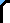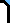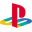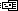Cheats DS GBA PC PS2 PS3 PSP Wii Xbox Xbox 360 New ReviewsSite Search

This Site WebCrash BandicootEmail this Page  |Printer Friendly Version

```Super Password:

This  Password  unlocks  all the  levels and gives you all the  hidden
items. Enter it at the "Password" screen:

------------------------------------------------------------------------
Triangle, Triangle, Triangle, Triangle, X, Square, Triangle, Triangle,
Triangle, Triangle,  Square,  X, Triangle, Circle, Triangle, Triangle,
Triangle, Circle, Square, Triangle, X, X, X, X

Debug menu:
At the Naughty Dog logo, hold Up+X until the title screen appears.
Then, hold LEFT+Square+O+Square+start. Now while playing ,you will
hear the sound of a snort. The game will resume. Press select to
display a debug menu.

Clearing obstacles:
On levels such as "Road to Nowhere", you can jump onto the ropes on
the side and easily clear most of the obstacles. To keep from falling
off the rope, you need to jump forward repeatedly on the rope

Secret levels:
* Look for a secret level at the top of the Native Fortress area. This
path runs behind the normal path and allows you to avoid enemies.
* In the Rolling Stones level, collect three Dr. Neo Cortex heads. One
is in a crate you must head-butt at the beginning of the level. The
second is in a cluster of dynamite boxes in the middle of the level,
and the third is by the exit warp. Get all three heads and you'll be
taken to a difficult bonus area where you must cross crates of TNT!

Enemy strategies:
* Don't use spin attacks against Papu Papu. Jump on his head after he
swings and missed you with his club.
* To defeat Ripper Roo, dodge him by moving between the front three
cubes, then when he approaches from the back, activate the TNT and
move away quickly.

Tips:
* Collect three tiki-mask power-ups and you will become invincible
for a short time.
* When outrunning the boulders, try to run in a straight line, and
follow the trails of fruit, as they will lead you around obstacles.
* Smashing all the boxes without dying will give you a "perfect"
screen and a gem.
* In the Cortex Power level, jump to the right and bounce off the
wall to avoid the spiked robots.
* When spin-busting crates on platforms, be sure you're standing
still before hitting the spin attack button, or you may spin right
off.

Super code to get to the third island:
Go to the password screen and enter the following:
Triangle, Triangle, Triangle, Triangle, Circle, X, Triangle,
Triangle, X, X, Triangle, Circle, Square, Circle, X, Triangle,
Triangle, Circle, Square, X, X, Square, Triangle, Triangle.

Access all levels:
This code lets you to access all 32 levels completed with all gems
and keys, allowing you to play the different endings:
Triangle, Triangle, Triangle, Triangle, X, Square, Triangle,
Triangle, Triangle, Triangle, Square, X, Triangle, Circle, Triangle,
Triangle, Triangle, Circle, Square, Triangle, X, X, X, X.

Posses all the keys and gems:
This code allows you to instantly have all the gems and keys in the
game:
Triangle, Triangle, Triangle, Triangle, X, Square, Triangle,
Triangle, Triangle, Triangle, Square, X, Triangle, Circle, Triangle,
Triangle, Triangle, Circle, Square, Triangle, X, X, X, X.

31 lives:
At the password screen, press Triangle, Triangle, Triangle, Triangle,
Circle, X, Square, Triangle, Triangle, Triangle, Triangle, Square, X,
Triangle, Circle, Triangle, Triangle, Triangle, Circle, Square,
Triangle, X, X, X, X then go down to castle machinery. When you
start, jump on gem and float up to lives.

Hints:

Beating the Bosses:
Having some trouble? These tips and tricks should help.

Boss one: Papu Papu:
To do to beat him, dodge the large stick that he swings around. When
he's done swinging, jump on his head and that sends him backwards.
When he's falling back, spin him 3 times and he will be gone.

Boss two: Ripper Roo:
Detonate the dynamite boxes he lays down when he's on a nearby square.
Do this three times and move on.

Boss three: Koala Kong:
Dodge the four boulders he throws at you. When the fourth one lands
in front of you, spin attack the rock and it will hit Koala Kong. Do
this 4 times and move on.

Boss four: Pinstripe:
Keep your eyes on him the whole time. When he jumps off the table,
wait till he stops shooting then spin attack him. To avoid being shot,
hide behind the couches. Hit him like that three or four times and
he'll be on the ground.

Boss five: Dr. Nitrus Brio:
To beat this boss, dodge the red and green beaker bombs that he throws.
Jump on the green blobs that are on the floor and those will crash
into Brio. When he has thee energy bars left, he turns into a demon.
Jump from the platform and smack his head. Repeat till he's on the
floor.

Final boss: Dr. Neo Cortex:
To beat the doc, watch his red and blue attacks. When you see a green
one, send it back. Remember, TIMING IS EVERYTHING.

Passwords:

% Complete - Password
2% Circle, Square, Circle, Square, Circle, Circle, Triangle, Square
6% Circle, X, Square, Triangle, Circle, Square, Square, Triangle
11% Square, Square, X, Square, Square, Triangle, X, Triangle
13% X, Square, X, Circle, X, Square, Triangle, X
18% Square, Square, Triangle, X, Circle, Circle, X, Triangle
19% Triangle, Triangle, X, Triangle, Circle, Circle, Square, X,
Square, X, Circle, Square, Circle, Square, X, Triangle, Square, X, X,
Square, X, X, Triangle, Square
22% Circle, Square, Square, Circle, Circle, X, X, X
27% X, Circle, X, Circle, Circle, X, Square, Circle
31% Square, X, Triangle, Triangle, X, Square, X, Triangle
36% X, Circle, Circle, Circle, X, Square, X, Square
38% Square, Square, X, Triangle, X, Square, Circle, X
52% Triangle, Triangle, Circle, X, X, Square, Square, Circle, Square,
Triangle, Triangle, X, X, Circle, Circle, Square, Circle, Square,
Triangle, Square, X, X, X, Circle
56% Triangle, Triangle, Triangle, Triangle, Triangle, X, Square,
Square, Square, Triangle, Triangle, X, X, Triangle, Square, Circle,
Triangle, Square, Circle, X, Circle, Circle, Triangle, X
57% Triangle, Triangle, Triangle, Square, Square, Square, Square,
Circle, Square, Triangle, Triangle, X, X, Triangle, Triangle, X,
Circle, Square, Square, X, Square, Triangle, X, Circle
63% Triangle, Triangle, X, X, X, X, Triangle, Square, Square, X, X, X,
Circle, Square, Triangle, Triangle, X, Circle, X, Circle, Triangle,
Triangle
68% Triangle, Triangle, X, X, Square, Circle, Square, Triangle,
Triangle, X, X, X, Circle, X, Square, Square, Triangle, Triangle,
Circle, Circle, Square, X, Circle

```